# 【算法练习五】逆波兰表达式

563+×3÷1-      ，    3421+÷2××3-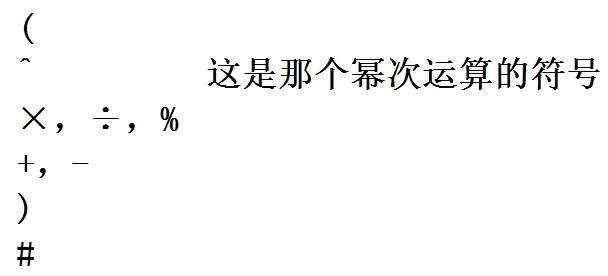5×(6+3)÷3-1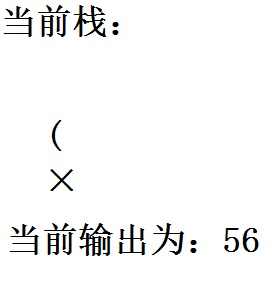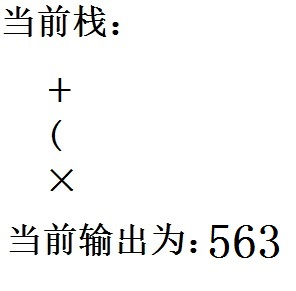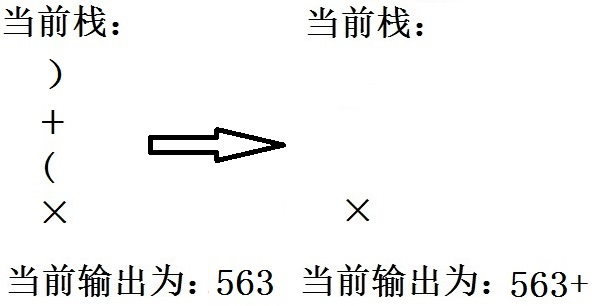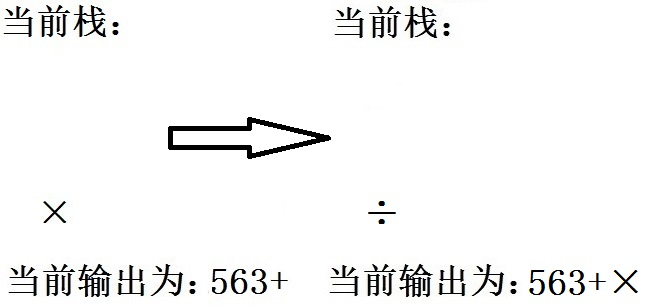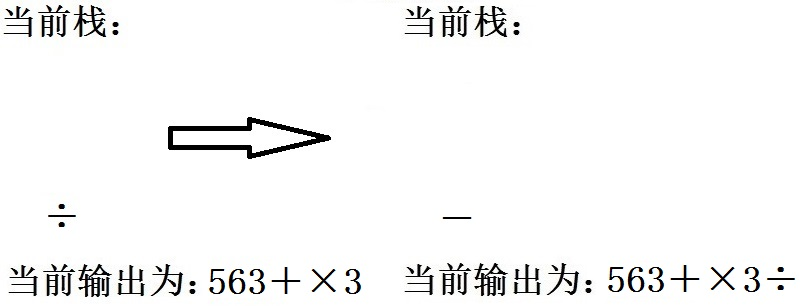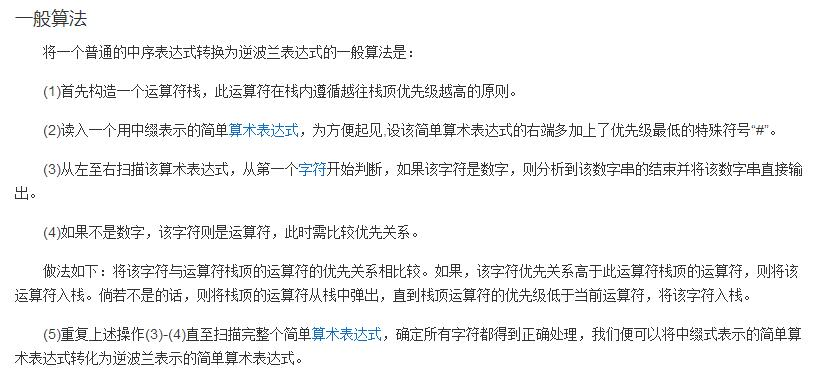#include<stdio.h>
#include<stdlib.h>
#include<string.h>
#define OK 1
#define ERROR 0
const int maxn = 20;

typedef struct Stack{
int max_size, top_index;
char *elements;
}Stack;

void init(Stack *s, int size){
s->max_size = size;
s->top_index = -1;
s->elements = (char*)malloc(sizeof(char)*size);
}

int push(Stack *s, char value){
if (s->top_index >= s->max_size – 1){
return ERROR;
}
s->top_index++;
s->elements[s->top_index]=value;
return OK;
}

void pop(Stack *s){
if (s->top_index <0){
return;
}
s->top_index–;
}

int top(Stack *s){
return s->elements[s->top_index];
}

void clear(Stack *s){
free(s->elements);
free(s);
}

int isOperator(char c){
return (c == ‘+’ || c == ‘-‘ || c == ‘*’ || c == ‘/’);
}

int priority(char c){
if (c == ‘*’ || c == ‘/’){
return 2;
}
if (c == ‘+’ || c == ‘-‘){
return 1;
}
else{
return 0;
}
}

int op(int a, int b, char c){
int res;
if (c == ‘+’){
res = a + b;
}
else if (c == ‘-‘){
res = a – b;
}
else if (c == ‘*’){
res = a*b;
}
else if (c == ‘/’){
res = a / b;
}
return res;
}

int main(){
char s[maxn];
scanf(“%s”, &s);
int n = strlen(s);
Stack *stack = (Stack*)malloc(sizeof(Stack));
init(stack, n);
char change[maxn];
int outlen = 0;
memset(change, ‘\0’, maxn);
memset(stack->elements, ‘\0’, n);
for (int i = 0; i<n; i++){
if (s[i] >= ‘0’&&s[i] <= ‘9’){
change[outlen++] = s[i];
}
while (isOperator(s[i])){
if (stack->top_index == -1 || priority(s[i])>priority(top(stack))){
push(stack,s[i]);
break;
}
else{
change[outlen++] = top(stack);
pop(stack);
}
}
}
while (stack->top_index != -1){
change[outlen++] = top(stack);
pop(stack);
}
for (int i = 0; i < sizeof(change); i++){
if (change[i] >= ‘0’&&change[i] <= ‘9’){
push(stack, change[i]);
}
else if (isOperator(change[i])){
char c = change[i];
int b = top(stack)-‘0’;
pop(stack);
int a = top(stack)-‘0′;
pop(stack);
push(stack, op(a, b, c)+’0’);
}
}
printf(“%s”,change);
printf(“\n%d”, top(stack)-‘0’);
clear(stack);
return 0;
}